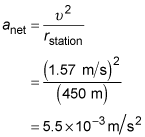##### Physics I: 501 Practice Problems For Dummies (+ Free Online Practice)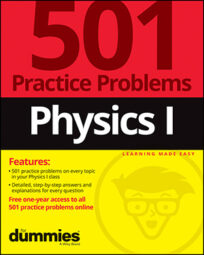Using physics, you can compare the acceleration due to gravity of two different revolving objects. For example, you can compare one planet to another, based on their respective masses and radii.

Here are some practice questions that illustrate this concept.

## Practice questions

1. Researchers at NASA load a 100-kilogram package onto a rocket on Earth. When the rocket lands on the surface of Neptune, where the acceleration of gravity is approximately 1.2 times as great as that on Earth, what will be the package's mass, rounded to the nearest integer?

2. How many times greater is the acceleration due to gravity at Jupiter's "surface" than at Earth's?

Use the following data and round your answer to the nearest integer.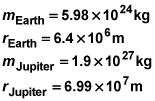3. A circular space station in the shape of a bicycle wheel has a mass of 1,800 kilograms. It rotates about a central axis 450 meters away from the station's outer ring and takes 30 minutes to make one revolution. What is the acceleration of an object located on the outer ring?

The following are the answers to the practice questions:

1. 100 kg

Although the weight of (the force of gravity on) the package differs on the two planets' surfaces, the mass does not differ. The mass of an object stays constant regardless of the forces acting upon it.

2. 3

The phrase "how many times greater" indicates that you need to use a ratio to solve this question. You need to know the ratio of Jupiter's gravitational acceleration compared with Earth's: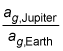Use the relationship that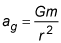where m is the mass of the particular planet and r is the radius of the planet: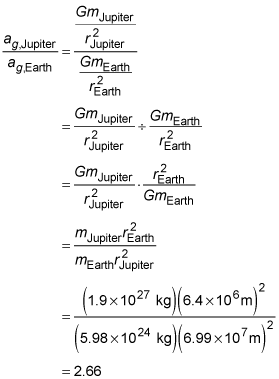Therefore, the acceleration of gravity at Jupiter's surface is 2.66 (or 3, rounded) times as great as that at Earth's surface.

3.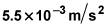You can figure out the acceleration of the object on the outer ring from the tangential velocity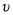and the radius of the ring: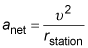Note that the tangential velocity of a point on the outer ring is currently the only value you don't have at your immediate disposal, so use the relationship between distance traveled (in this case, the circumference of the circle made by the object in one rotation of the station) and the time it takes to travel that distance (30 minutes):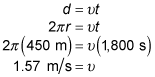Recall that the circumference of a circle C is equal to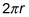and convert the time to the desired units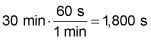Now you can substitute everything into the formula you derived earlier to calculate the acceleration: